## 3. 2. 1 Secondary Graph Method - Gauß Integral Theorem

As a first step a function space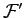is defined on the tessellated simulation domain. Typically, linear shape functions are assumed, however, it only has to be assured that all shape functions are continuous . For the secondary graph simulation method only vertices and edges of the cell complex are used. Furthermore, the cell complex is associated its dual graph comprising the cells of the Voronoi tesselation as well as the boundary surfaces of these boxes. Dual elements of vertices are cells which cover the set of points closer to the respective vertex than to any other vertex . Each edge is assigned a part of the boundary surface of the dual cell. If the original tesselation fulfills the Delauney property, a dual graph can be constructed in a unique manner and the cells are disjoint and bounded by the surfaces. If the Delauney property holds true for a cell complex, each vertexis assigned a dual cell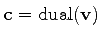. Consequently, an edge incident with the vertexbounds the cell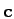.

Each local shape function is defined with respect to a vertex and is non-zero on all cells incident with this vertex. In the finite volume method, the governing differential operator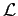has the form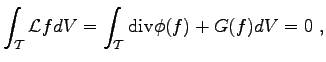(3.21)

where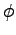is a vector valued and depends on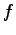and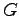is an ordinary function. The equation is re-written under the Gauß integral theorem and yields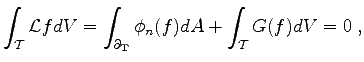(3.22)

where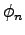denotes the normal component of the resulting vector of the functional. A first approach for the formulation of finite volume schemes is based on the assumption that for each vertex the local shape function defined on the incident cells is evaluated by calculating an integral on the surface of the dual cell. The part of the boundary of the dual cell of the vertexwhich is in a cellis used as integration domain.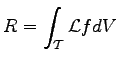(3.23)(3.24)

where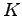denotes the following integral formula. The function value within the cell (and therefore also on the dual surface) depends on all quantity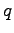values associated with a vertex incident to the respective cell.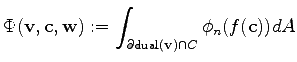(3.25)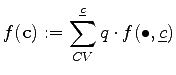(3.26)

The function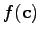denotes the value of the solution function within the respective cell of evaluation,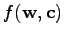denote the cell based shape functions of the function space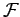.

Michael 2008-01-16